Home Practice
For learners and parents For teachers and schools
Textbooks
Full catalogue
Pricing SupportLog in

We think you are located in United States. Is this correct?

# 11.2 Electrical machines - generators and motors

## 11.2 Electrical machines - generators and motors (ESCQ4)

We have seen that when a conductor is moved in a magnetic field or when a magnet is moved near a conductor, a current flows in the conductor. The amount of current depends on:

• the speed at which the conductor experiences a changing magnetic field,
• the number of coils that make up the conductor, and
• the position of the plane of the conductor with respect to the magnetic field.
The effect of the orientation of the conductor with respect to the magnetic field is illustrated in Figure 11.1.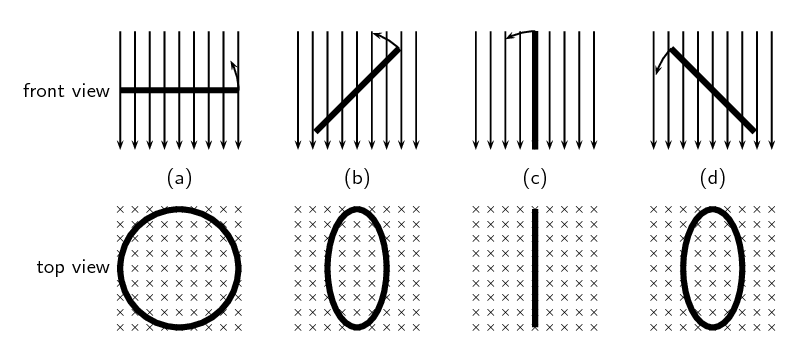Figure 11.1: Series of figures showing that the magnetic flux through a conductor is dependent on the angle that the plane of the conductor makes with the magnetic field. The greatest flux passes through the conductor when the plane of the conductor is perpendicular to the magnetic field lines as in Figure 11.1 (a). The number of field lines passing through the conductor decreases, as the conductor rotates until it is parallel to the magnetic field Figure 11.1 (c).

If the emf induced and the current in the conductor were plotted as a function of the angle between the plane of the conductor and the magnetic field for a conductor that has a constant speed of rotation, then the induced emf and current would vary as shown in Figure 11.2. The current alternates around zero and is known as an alternating current (abbreviated AC).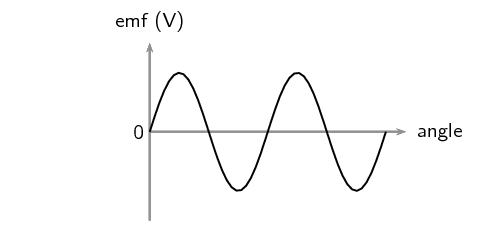Figure 11.2: Variation of induced emf and current as the angle between the plane of a conductor and the magnetic field changes.

The angle changes as a function of time so the above plots can be mapped onto the time axis as well.

The emf, $$\mathcal{E}$$, induced around a single loop of conductor is proportional to the rate of change of the magnetic flux, φ, through the area, $$A$$, of the loop. This can be stated mathematically as:

$\mathcal{E} =-N\frac{\Delta \phi }{\Delta t}$

where $$\phi =B·A\cos\theta$$ and $$B$$ is the strength of the magnetic field.

Faraday's Law relates induced emf to the rate of change of magnetic flux, which is the product of the magnetic field strength and the cross-sectional area the field lines pass through. The cross-sectional area changes as the loop of the conductor rotates which gives rise the $$\cos\theta$$ factor. $$\theta$$ is the angle between the normal to the surface area of the loop of the conductor and the magnetic field. As the closed loop conductor changes orientation with respect to the magnetic field, the amount of magnetic flux through the area of the loop changes and an emf is induced in the conducting loop.

temp text

### AC generator (ESCQ6)

The principle of rotating a conductor in a magnetic field to generate current is used in electrical generators. A generator converts mechanical energy (motion) into electrical energy.

Generator

A generator is a device that converts mechanical energy into electrical energy.

The layout of a simple AC generator is shown in Figure 11.3. The conductor is formed of a coil of wire, placed inside a magnetic field. The conductor is manually rotated within the magnetic field. This generates an alternating emf. The alternating current needs to be transmitted from the conductor to the load, which is the system requiring the electrical energy to function.

The load and the conductor are connected by a slip ring. A slip ring is a connector which is able to transmit electricity between rotating portions of a machine. It is made up of a ring and brushes, one of which is stationary with respect to the other. Here, the ring attaches to the conductor and the brushes are attached to the load. Current is generated in the rotating conductor, passes into the slip rings, which rotate against the brushes. The current is transmitted through the brushes into the load, and the system is thus powered.

The direction of the current changes with every half turn of the coil. As one side of the loop moves to the other pole of the magnetic field, the current in the loop changes direction. This type of current which changes direction is known as alternating current and Figure 11.4 shows how it comes about as the conductor rotates.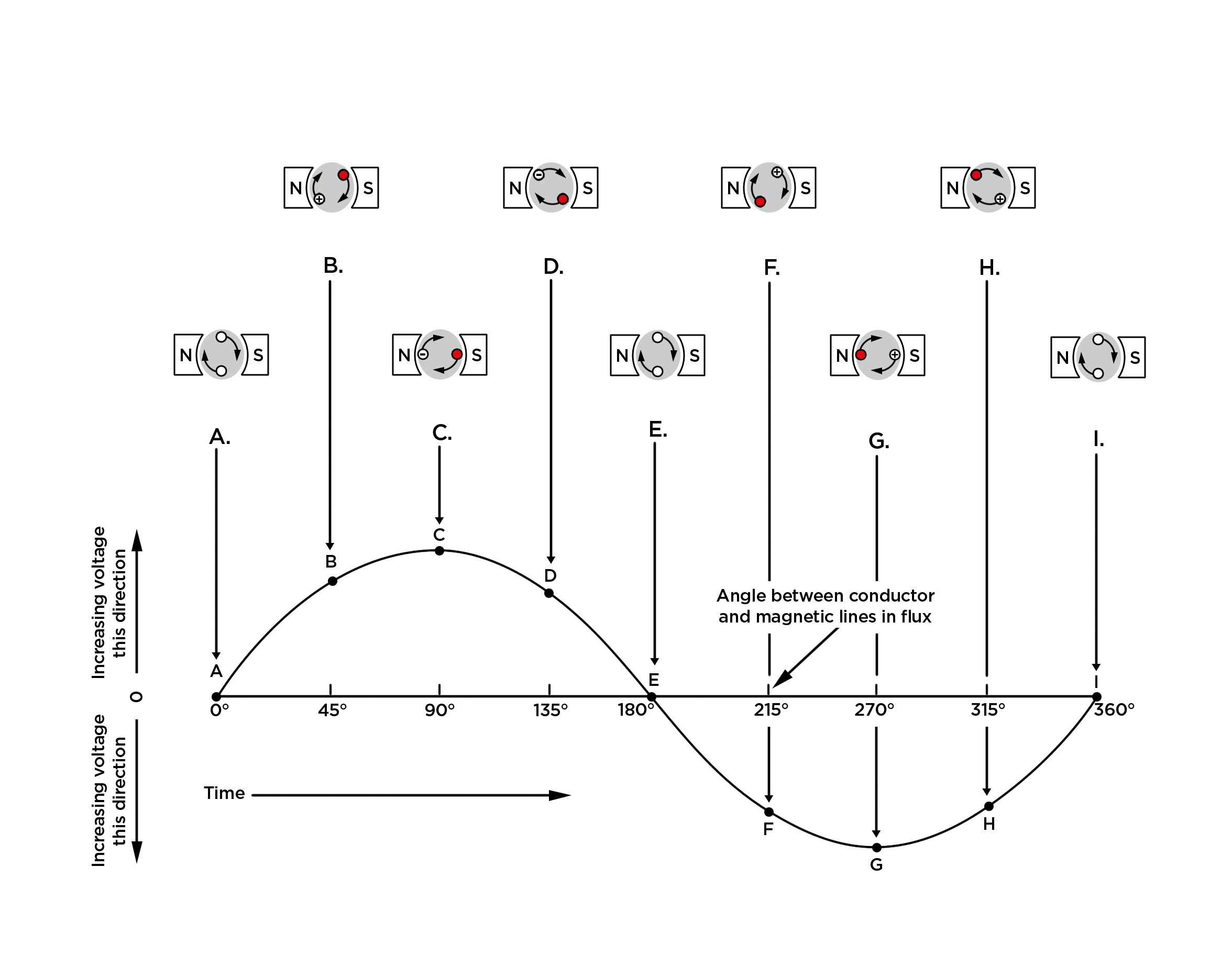Figure 11.4: The red (solid) dots represent current coming out of the page and the crosses show current going into the page.

AC generators are also known as alternators. They are found in motor cars to charge the car battery.

temp text

### DC generator (ESCQ7)

A simple DC generator is constructed the same way as an AC generator except that there is one slip ring which is split into two pieces, called a commutator, so the current in the external circuit does not change direction. The layout of a DC generator is shown in Figure 11.5. The split-ring commutator accommodates for the change in direction of the current in the loop, thus creating direct current (DC) current going through the brushes and out to the circuit. The current in the loop does reverse direction but if you look carefully at the 2D image you will see that the section of the split-ring commutator also changes which side of the circuit it is touching. If the current changes direction at the same time that the commutator swaps sides the external circuit will always have current going in the same direction.

The shape of the emf from a DC generator is shown in Figure 11.6. The emf is not steady but is the absolute value of a sine/cosine wave.

### AC versus DC generators (ESCQ8)

The problems involved with making and breaking electrical contact with a moving coil are sparking and heat, especially if the generator is turning at high speed. If the atmosphere surrounding the machine contains flammable or explosive vapours, the practical problems of spark-producing brush contacts are even greater.

If the magnetic field, rather than the coil/conductor is rotated, then brushes are not needed in an AC generator (alternator), so an alternator will not have the same problems as DC generators. The same benefits of AC over DC for generator design also apply to electric motors. While DC motors need brushes to make electrical contact with moving coils of wire, AC motors do not. In fact, AC and DC motor designs are very similar to their generator counterparts. The AC motor is depends on the reversing magnetic field produced by alternating current through its stationary coils of wire to make the magnet rotate. The DC motor depends on the brush contacts making and breaking connections to reverse current through the rotating coil every 1/2 rotation (180 degrees).

temp text

### Electric motors (ESCQ9)

The basic principles of operation for an electric motor are the same as that of a generator, except that a motor converts electrical energy into mechanical energy (motion).

Electric motor

An electric motor is a device that converts electrical energy into mechanical energy.

If one were to place a moving charged particle in a magnetic field, it would experience a force called the Lorentz force.

The Lorentz Force

The Lorentz force is the force experienced by a moving charged particle in an electric and magnetic field. The magnetic component is:

$F=qvB$

where $$F$$ is the force (in newtons, N), $$q$$ is the electric charge (in coulombs, C), $$v$$ is the velocity of the charged particle (in $$\text{m·s^{-1}}$$) and $$B$$ is the magnetic field strength (in teslas, T).

The force on a current-carrying conductor due to a magnetic field is called Ampere's law.

The direction of the magnetic force is perpendicular to both the direction of the flow of current and the direction of the magnetic field and can be found using the Right Hand Rule as shown in the picture below. Use your right hand; your first finger points in the direction of the current, your second finger in the direction of the magnetic field and your thumb will then point in the direction of the force.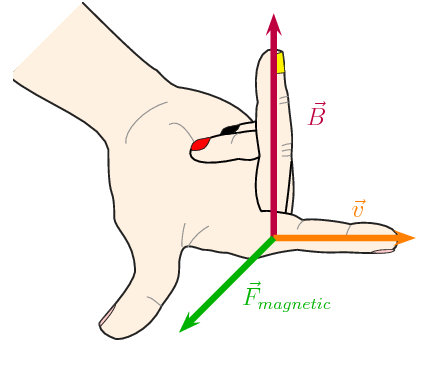Both motors and generators can be explained in terms of a coil that rotates in a magnetic field. In a generator the coil is attached to an external circuit that is turned, resulting in a changing flux that induces an emf. In a motor, a current-carrying coil in a magnetic field experiences a force on both sides of the coil, creating a twisting force (called a torque, pronounce like 'talk') which makes it turn.

If the current is AC, the two slip rings are required to create an AC motor. An AC motor is shown in Figure 11.7

If the current is DC, split-ring commutators are required to create a DC motor. This is shown in Figure 11.8.

temp text

### Real-life applications (ESCQB)

#### Cars

A car contains an alternator. When the car's engine is running the alternator charges its battery and powers the car's electric system.

## Alternators

Try to find out the different current values produced by alternators for different types of machines. Compare these to understand what numbers make sense in the real world. You will find different values for cars, trucks, buses, boats etc. Try to find out what other machines might have alternators.

A car also contains a DC electric motor, the starter motor, to turn over the engine to start it. A starter motor consists of the very powerful DC electric motor and starter solenoid that is attached to the motor. A starter motor requires a very high current to crank the engine and is connected to the battery with large cables to carry large current.

#### Electricity generation

In order to produce electricity for mass distribution (to homes, offices, factories and so forth), AC generators are usually used. The electricity produced by massive power plants usually has a low voltage which is converted to high voltage. It is more efficient to distribute electricity over long distances in the form of high voltage power lines.

The high voltages are then coverted to 240 V for consumption in homes and offices. This is usually done within a few kilometres of where it will be used.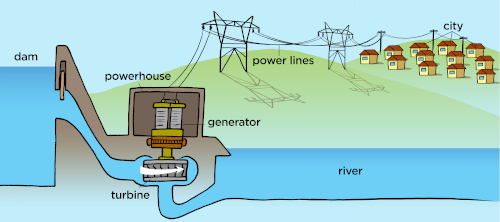Figure 11.9: AC generators are used at power plants (all types, hydro- and coal-plants shwon) to generate electricity.

## Generators and motors

Textbook Exercise 11.1

State the difference between a generator and a motor.

An electrical generator is a mechanical device to convert energy from a source into electrical energy.

An electrical motor is a mechanical device to convert electrical energy from a source into another form energy.

Use Faraday's Law to explain why a current is induced in a coil that is rotated in a magnetic field.

Faraday's law says that a changing magnetic flux can induce an emf, when the coil rotates in a magnetic field it is possible for the rotation to change the flux thereby inducing an emf.

If the rotation of the coil is such that the flux doesn't change, i.e. the surface of the coil remains parallel to the magenetic field, then there will be no induced emf.

Explain the basic principle of an AC generator in which a coil is mechanically rotated in a magnetic field. Draw a diagram to support your answer.

Solution not yet available

Explain how a DC generator works. Draw a diagram to support your answer. Also, describe how a DC generator differs from an AC generator.

Solution not yet available

Explain why a current-carrying coil placed in a magnetic field (but not parallel to the field) will turn. Refer to the force exerted on moving charges by a magnetic field and the torque on the coil.

A current-carrying coil in a magnetic field experiences a force on both sides of the coil that are not parallel to the magnetics field, creating a twisting force (called a torque) which makes it turn. Any coil carrying current can feel a force in a magnetic field. The force is due to the magnetic component of the Lorentz force on the moving charges in the conductor, called Ampere's Law. The force on opposite sides of the coil will be in opposite directions because the charges are moving in opposite directions.

Explain the basic principle of an electric motor. Draw a diagram to support your answer.

Solution not yet available

Give examples of the use of AC and DC generators.

Cars (both AC and DC), electricity generation (AC only), anywhere where a power supply is needed.

Give examples of the uses of motors.

Pumps, fans, appliances, power tools, household appliances, office equipment.